# Kirchhoff's Junction Rule Formula

Kirchhoff's Junction Rule Formula

In a closed circuit, there can be any number of circuit elements, such as batteries and resistors. The circuit can branch, creating "junctions", where the circuit separates or recombines. The sum of the currents in and out of a circuit junction must be zero. This is known as Kirchhoff's Junction Rule. Current is measured in Amperes (A).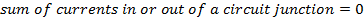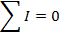I = current, (Amperes, A)

Kirchhoff's Junction Rule Formula Questions:

1) The circuit in the figure below consists of two resistors and a voltage source (battery). The current before junction "a" is Ia, the current through resistor R1 is I1, and the current through resistor R2 is I2. Values are given in the figure for Ia and I2. Based on this figure, what is the value of current I1?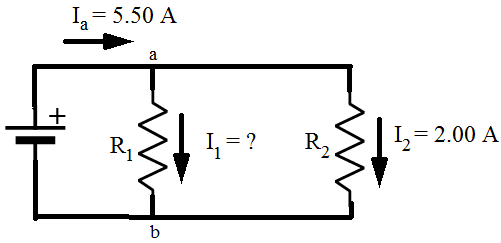Answer: Kirchhoff's Junction Rule states that the sum of the currents in and out of a junction must be equal to zero. In this case, I1 is connected to junction "a", and the sum of the currents in and out of junction "a" can be used to find the value of I1. The direction of the currents at the junction are important. In this case, current is shown flowing through the circuit in a clockwise direction. This means that there is one current flowing in, and two currents flowing out of junction "a". The sum of the currents in and out of junction "a" is: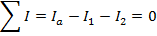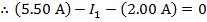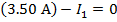The value of I1 can be found by rearranging the formula above:The value of current I1 is 3.50 A(Amperes).

2) The circuit in the figure below consists of three resistors and a voltage source (battery). The current before junction "a" is Ia, the current before junction "b" is Ib, the current through resistor R1 is I1, the current through resistor R2 is I2 , and the current through resistor R3 is I3. Values are given in the figure for Ia, I1, and I2. Based on this figure, what is the value of current I3?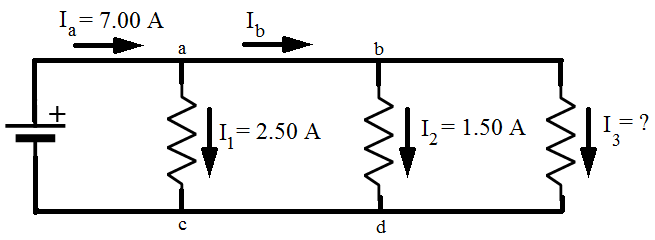Answer: Kirchhoff's Junction Rule states that the sum of the currents in and out of a junction must be equal to zero. In this case, I3 is connected to junction "b". The directions of the currents at the junctions are important. In this case, current is shown flowing through the circuit in a clockwise direction. The sums of the currents flowing in and out of junctions "a" and "b" can be used to find the value of I3. The sum of the currents in and out of junction "a" is: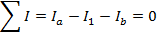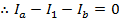The sum of the currents in and out of junction "b" is: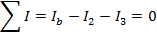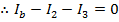These two equations can be combined to solve for I3. The common way to express this is that we have "two equations and two unknowns". The values of Ib and I3 are unknown, but with two equations, there is enough information to solve the problem. The equations can be labeled (1) and (2):

(1)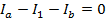(2)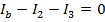Equation (1) can be rearranged to isolate Ib on the left side of the equal sign: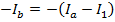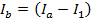Now, this equation for Ib can replace Ib in equation (2):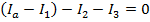This can now be rearranged to solve for I3: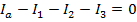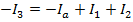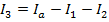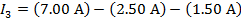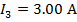The value of current I3 is 3.00 A(Amperes).